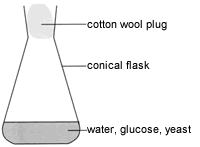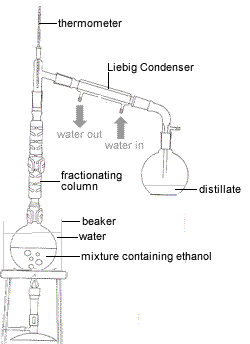Fermentation Chemistry Tutorial

Key Concepts

• Fermentation of fermentable sugars by a suitable yeast produces ethanol (ethyl alcohol).
• Fermentable sugars are:
⚛ glucose (also known as dextrose, blood sugar, or grape sugar), C6H12O6

⚛ mannose, C6H12O6

⚛ galactose, C6H12O6

⚛ fructose (also known as levulose), C6H12O6

• Yeast is a single celled fungus containing a number of enzymes useful during fermentation.
Brewers yeast and wine yeast, for example, contain zymase, an enzyme that can convert glucose to ethanol.
• The overall chemical equation for the fermentation of a fermentable sugar such as glucose by an enzyme such as zymase is:

 glucose zymase → ethanol + carbon dioxide gas C6H12O6(aq) zymase → 2C2H5OH(aq) + 2CO2(g)

• The reaction conditions for fermentation are:
⚛ aqueous environment
(aqueous solutions)

⚛ warm temperature
(different enzymes will have different optimum temperatures)

⚛ anaerobic environment
(no oxygen present)

⚛ slightly acidic pH

• Fermentation will cease if
⚛ the ethanol concentration becomes too great
(about 5% by volume ethanol for brewers yeast, about 14% by volume ethanol for wine yeast)

⚛ the temperature is high enough to kill the yeast
(above approximately 27°C for brewers yeast)

⚛ the pH falls below ≈6

• Distillation of the aqueous mixture produced from fermentation can produce a solution containing approximately 95% by volume ethanol.
• The fermentation of plant sugars is used to produce alcoholic beverages such as wine and beeer.
• Fermentation can also be used to produce ethanol (ethyl alcohol) which can be used as a fuel.
Ethanol produced by fermentation and used as a fuel is referred to as a:
(a) renewable fuel (plants can be re-grown)
(b) biofuel, or biomass fuel, (fuel obtained from living organisms)

No ads = no money for us = no free stuff for you!

Preparation of Ethanol by Fermentation of Glucose in the Laboratory

Producing ethanol of a high concentration is a two-step process:

1. Fermentation of glucose to produce the ethanol (ethyl alcohol)
2. Distillation of the fermentation products to purify the ethanol (ethyl alcohol)

Do you know this?

Play the game now!

Step 1: Fermentation of Glucose to Produce Ethanol

The procedure used to ferment glucose in the school laboratory is set out below:1. Glucose is dissolved in warm water in a conical flask.
2. Some yeast is added to the glucose solution and cotton wool is used to plug the top of the conical flask.
3. The flask is left in a warm place to allow fermentation to take place.
4. When bubbles of carbon dioxide are no longer forming, the fermentation reaction has ceased.

The chemistry of the fermentation of glucose to produce ethanol is quite complicated.
A simplified outline is given below.

The first step in the fermentation reaction is the conversion of glucose (C6H12O6) to pyruvic acid (CH3-CO-COOH) using the hydrogen carrier NADH present in the yeast (1):

Glucose has been oxidised, oxygen has been gained to produce pyruvic acid.

Zymase, an enzyme in the yeast, converts the pyruvic acid to ethanol and carbon dioxide using the hydrogen carrier NADH:

Pyruvic acid has lost oxygen to produce ethanol so it has been reduced.

So, the overall equation for the fermentation of glucose is:

 C6H12O6 + 2NAD+ → 2CH3-CO-COOH + 2NADH + 2H+ 2CH3-CO-COOH + 2NADH + 2H+ → 2C2H5OH + 2CO2 + 2NAD+ C6H12O6 → 2C2H5OH + 2CO2

In reality, the chemistry of fermentation is actually much more complex than this, with the glucose being incorporated into other by-products such as pyruvic acid, acetaldehyde and glycerol.

Do you understand this?

Take the test now!

Distillation of Fermentation Products to Produce Ethanol

Distillation is a technique that separates a mixture of different compounds with different boiling points.
At a pressure of 1 atmosphere (or 101.3kPa) the boiling point of pure water is 100°C while the boiling point of ethanol is 78.3°C.
Because ethanol and water have different boiling points we can separate a mixture of ethanol and water by boiling it.
Ethanol, with the lower boiling point, will boil first. We can condense the hot vapor using a water-cooled condenser so that the liquid ethanol can be collected in a separate vessel.
Once all the ethanol has boiled off, the temperature of the solution will start increasing again until the water starts to boil off at 100°C.
We stop collecting the liquid as soon as the temperature of the solution starts to increase so that we don't collect water in the same vessel that contains our ethanol!

The procedure for distilling your ethanol/water mixture is set out below:1. The mixture containing ethanol is placed in a round-bottomed flask or Claisen flask.
2. Since ethanol is flammable and has a boiling point below that of water, the flask is placed in a water bath to gently heat the mixture.
3. Using a fractionating column reduces the number of distillations required to complete the separation of the ethanol as it provides a continuous series of partial condensations of the vapour and partial vaporisations of the condensate.
4. A thermometer is used to ensure that only the fraction that boils at 78.3°C is collected as this is the ethanol fraction.
5. The ethanol vapor entering the Liebig condenser (water-cooled condenser) is cooled and condenses to liquid ethanol.
One end of a flexible tube is connected to the bottom of the Liebig condenser and the other end is connected to a water tap.
One end of a flexible tube is connected the top of the Liebig condenser and the other end of this tube is placed in the sink.
When the tap is turned on, water flows into the bottom of the Liebig condenser and out of the top of the Liebig condenser.
This constant stream of cool water allows heat to be transferred from the hot vapour in the Liebig condenser to the cooler water which is flowing away, thereby condensing the vapour to the liquid.
6. The product of the distillation is called the distillate and is collected in a suitable clean, dry flask (often a conical flask also known as an Erlenmeyer flask).
The distillate will contain ethanol as long as the temperature of the vapour as measured by the thermometer is 78.3oC.
When the temperature of the vapour begins to rise, the flask containing the distillate should be removed and replaced with a clean flask to prevent contamination of the ethanol distillate, and the bunsen burner turned off.

In practice, it is not possible to obtain pure 100% ethanol using this distillation method because the constant boiling point mixture collected as the distillate contains about 95% ethanol and 5% water. (3)
Further concentration of the ethanol is possible using other separation techniques such as vacuum distillation, or, by adding another substance such as benzene to the mixture.

Can you apply this?

Join AUS-e-TUTE!

Take the exam now!

Worked Example of a Fermentation Problem

Problem Solving using the StoPGoPS approach.

 Question: Chris the Chemist has been asked to produce 100 mL of ethanol by fermenting glucose using zymase containing yeast as described on this page above. If the density of ethanol is 0.79 g mL-1, what minimum mass of glucose must Chris use assuming complete conversion of glucose to ethanol?

STOP STOP! State the Question.
What is the question asking you to do?
Determine the mass of glucose

m(glucose) = ? g

PAUSE PAUSE to Prepare a Game Plan
(1) What information (data) have you been given in the question?
reactant: glucose (C6H12O6)

product: ethanol (C2H5OH)

volume of ethanol = 100 mL

density of ethanol = 0.79 g mL-1

(2) What is the relationship between what you know and what you need to find out?

(a) Write a balanced chemical equation for the reaction:
glucose → ethanol + carbon dioxide

(b) Calculate mass of ethanol:
mass ethanol = density (g mL-1) × volume (mL)

(c) Calculate moles of ethanol:
moles ethanol = mass(ethanol) ÷ molar mass(ethanol)

(d) Calculate moles of glucose:
mole ratio (stoichiometric ratio) ethanol:glucose

(e) Calculate mass of glucose:
mass(glucose) = mole(glucose) × molar mass(glucose)

GO GO with the Game Plan

(a) Write a balanced chemical equation for the reaction:
 glucose zymase → ethanol + carbon dioxide C6H12O6 zymase → 2C2H5OH + 2CO2(g)

(b) Calculate mass of ethanol:
mass ethanol = density (g mL-1) × volume (mL)
mass(ethanol) = 0.79 g mL-1 × 100 mL = 79 g

(c) Calculate moles of ethanol:
moles ethanol = mass(ethanol) ÷ molar mass(ethanol)
moles(ethanol) = 79 g ÷ (2 × 12.01 + 6 × 1.008 + 16.00)g mol-1 = 79 ÷ 46.068 mol-1 = 1.7 mol

(d) Calculate moles of glucose:
mole ratio (stoichiometric ratio) ethanol:glucose is 2:1
or ethanol:glucose is 1:½
1 mole of ethanol is produced by ½ mole of glucose
So, 1.7 moles of ethanol is produced by ½ × 1.7 mol = 0.85 moles of glucose

(e) Calculate mass of glucose:
mass(glucose) = mole(glucose) × molar mass(glucose)
mass(glucose) = 0.85 mol × (6 × 12.01 + 12 × 1.008 + 6 × 16.00) g mol-1 = 0.85 × 180.156 g-1 = 153 g

mass of glucose = 153 g

PAUSE PAUSE to Ponder Plausibility

Work backwards using the calculated value of mass of glucose to determine the maximum volume of ethanol that could be obtained.

C6H12O6 → 2C2H5OH + CO2
n(C6H12O6) = mass ÷ molar mass ≈ 153 g ÷ 180 = 0.85 mol
n(C2H5OH) = 2 × n(C6H12O6) = 2 × 0.85 = 1.7 mol
m(C2H5OH) = moles × molar mass = 1.7 mol × 46 g/mol = 78 g
V(C2H5OH) = mass ÷ density = 78 ÷ 0.79 = 99 mL

Since the value of 99 mL for the volume of ethanol is close to 100 mL, we are reasonably confident that our mass of glucose is correct.

STOP STOP! State the Solution
minimum mass of glucose needed is 153 g

Can you apply this?

Join AUS-e-TUTE!

Take the exam now!

Footnotes:

(1) NAD+ is Nicotinamide Adenine Dinucleotide. It is a biological oxidizing agent.

(2) In biological systems we often think about oxidation as a gain of oxygen (or a loss of hydrogen).
Reduction can then be thought of as a loss of oxygen (or a gain of hydrogen).

(3) Ethanol and water form an azeotropic mixture with a minimum boiling point of 78.3°C at 1 atm pressure.